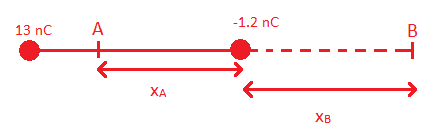# At what point or points on the x-axis is the electric potential zero?

## Homework Statement

A 13.0 nC charge is at x= 0 cm and a -1.2 nC charge is at 6 cm.

At what point or points on the x-axis is the electric potential zero?

V=kq/r

## The Attempt at a Solution

0=13K/r + 1.2K/(r-0.06)
13(r-0.06)=-1.2r
r=5.5 cm

There is another point where the electric potential is zero. I have tried r+0.06 and moving the (r-0.06) to the other side of the equation, but I haven't found a correct second point. How do I find the second point? Thanks!

Hi smoicsObviously the point with V=0 will be be near 1.2 nC charge

SO let the points be A and B
now just write the eqn for the two points and find xA and xB

#### Attachments

Last edited:
I don't understand why you're putting point A where it is. Am I going to have 2 variables in one equation? Or are you using 2 equations?

I don't understand why you're putting point A where it is. Am I going to have 2 variables in one equation? Or are you using 2 equations?

These are two different cases not 2 eqn's

Remember that electric potential is scalar and thus can also be zero betw. the charges

Edit: if you are talking about why i drew A closer to 13nC then its just my carelessness :tongue:

SammyS
Staff Emeritus
Homework Helper
Gold Member

## Homework Statement

A 13.0 nC charge is at x= 0 cm and a -1.2 nC charge is at 6 cm.

At what point or points on the x-axis is the electric potential zero?

V=kq/r

## The Attempt at a Solution

0=13K/r + 1.2K/(r-0.06)
13(r-0.06)=-1.2r
r=5.5 cm

There is another point where the electric potential is zero. I have tried r+0.06 and moving the (r-0.06) to the other side of the equation, but I haven't found a correct second point. How do I find the second point? Thanks!

The equation: $$0 = k\,\frac{13}{r}\,+\,k\,\frac{1.2}{r-0.06}$$

is equivalent to: $$0 = k\,\frac{13}{r}\,+\,k\,\frac{-1.2}{0.06-r}$$

This gives a result which is valid only between the two charges.

If a charge of 13 nC is at x = 0, and a charge of -1.2 nC is at x = 0.06 m, then the electric potential at x on the x-axis is given by:

$$V(x)=k\,\frac{13nC}{|x|}+k\,\frac{-1.2nC}{|0.06-x|}$$